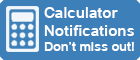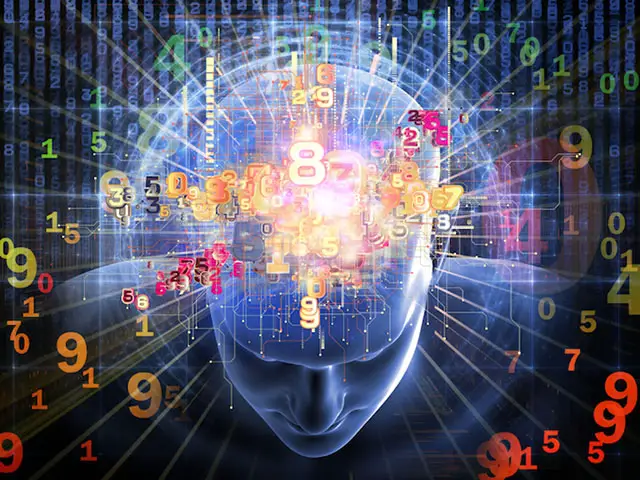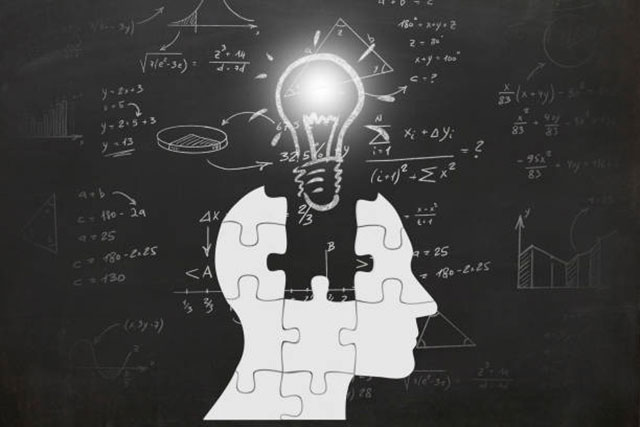Calculator Library
Free Online CalculatorsHome » 87 Calculators » Blog » Calculators and Mental Math Skills

Huge Domain Name Sale | Free Domains
Domain names on sale at crazy prices. Register a new domain for FREE when you buy another service.

## CALCULATORS AND MENTAL MATH SKILLSCalculators have become a ubiquitous tool in modern society, present in almost every classroom and office. However, what impact do these devices have on our mental math skills? While calculators have certainly made performing complex calculations easier, there's a growing concern among educators and experts that they may be reducing our ability to perform mental math.

The use of calculators has been on the rise for decades, with students of all ages using them to complete math homework and take tests. However, research suggests that relying on calculators may be detrimental to our mental math abilities. Studies have shown that students who use calculators regularly perform worse on math tests that do not allow for calculator use than those who do not regularly use calculators. In this regard, an old joke calls calculators "weapons of math destruction"!

Additionally, experts argue that using calculators can lead to a lack of understanding of basic math concepts. Without the need to perform calculations mentally, students may not fully grasp math's underlying principles and mechanics. This can lead to a lack of fluency and proficiency in the subject, making it more difficult to apply math skills in real-world scenarios.

## What Is Mental Math?

Mental math, also known as mental arithmetic or "doing math in your head", is the process of solving mathematical problems without using paper and pencil or a calculator. It requires using memory, visualization and manipulation of mathematical concepts, and the ability to reason mathematically. The practice of mental math can be applied to various mathematical operations, including basic arithmetic, geometry, algebra and even calculus.

Mental math is a valuable skill that allows us to quickly and accurately perform calculations in our daily lives. It's beneficial when using paper and pencil, or a calculator isn't feasible, such as when making quick calculations in the grocery store, calculating tips at a restaurant or solving a math problem during a test or exam. The ability to perform mental math allows for a faster and more efficient problem-solving process, making it a valuable skill in personal and professional settings.

In addition to its practical applications, mental math also plays a vital role in improving problem-solving skills, logical reasoning and overall mathematical fluency. By regularly practicing mental math, individuals can improve their ability to understand and apply mathematical concepts, leading to better performance in mathematics-related subjects and careers. It also helps to develop cognitive skills such as memory, attention and concentration. Furthermore, it can help to improve the ability to think critically and make connections between mathematical concepts, which can help to develop a deeper understanding of math and increase the ability to apply mathematical skills in real-world scenarios.

## Studies On Calculator Use And Mental Math Abilities

Recent studies have found that there may be a correlation between calculator use and decreased mental math abilities. One study by researchers at the University of Cambridge found that students who regularly used calculators on math tests had lower scores than those who didn't. Additionally, a study published in the Journal of Educational Psychology found that students who were allowed to use calculators on math tests had a more challenging time solving math problems without using a calculator.

It's important to note that this doesn't necessarily mean that calculators are inherently evil; they can be handy tools when used correctly. However, it does suggest that over-reliance on calculators can lead to decreased mental math abilities.

## The Convenience Of Calculators

Calculators offer a level of convenience and ease of use that was previously unimaginable. With the press of a button, we can solve mathematical equations that would have taken hours to work out by hand. This convenience has led to the widespread use of calculators in classrooms and workplaces, as well as for personal use.

In classrooms, calculators are often used to help students understand mathematical concepts. They can be used to perform quick calculations and help students visualize mathematical relationships. Additionally, calculators are often used in standardized testing to ensure that all students have equal access to tools that can help them perform calculations.

In the workplace, calculators perform a wide range of calculations, from simple arithmetic to complex financial analysis. They can be used to calculate budgets, analyze data and perform other essential tasks that require mathematical calculations.

In personal use, calculators are used for everything from working out a tip at a restaurant to determining how much to save for retirement. They are essential tools for managing finances and making important decisions.

## The Downside Of Calculator Dependence

However, as we rely more heavily on calculators, this convenience has a downside. As we use calculators more frequently, we lose our mental math ability. Studies have shown that students who use calculators regularly perform worse on mental math tests than those who do not use calculators as frequently. Additionally, adults relying heavily on calculators may find it difficult to perform simple calculations when a calculator is unavailable.

This dependence on calculators can have serious consequences. For example, in a professional setting, an employee unable to perform basic calculations may struggle to perform their job effectively. Alternatively, in a personal environment, an individual unable to perform simple calculations may have difficulty managing their finances and making important decisions.

## The Impact Of Calculator Use On Learning

Calculator use also has an impact on how students learn mathematics. When students use calculators to perform calculations, they may not fully understand the mathematical concepts behind the equations they are solving. This can lead to a lack of understanding of basic mathematical concepts, making it difficult for students to solve more complex equations.

Additionally, when students can use calculators to perform calculations, they may not develop the important problem-solving skills necessary to understand mathematical concepts. They may become reliant on calculators to solve problems and may not be able to think critically and creatively when presented with a math problem.

## Strategies For Improving Mental Math Ability

To combat the negative effects of calculator dependence, it's essential to maintain and improve mental math skills. Here are a few strategies to consider:

• Limit calculator use: Try to solve mathematical equations using mental math whenever possible. This will help to maintain and improve mental math skills.
•Practice mental math exercises: There are many mental math exercises and games available online that can help to improve mental math abilities.
• Learn key mathematical concepts and formulas: Understanding the concepts and formulas behind mathematical equations can make it easier to solve them mentally.
• Incorporate mental math into everyday life: Incorporate mental math into daily activities such as shopping, cooking and budgeting. This can help to make mental math more natural and intuitive.
• Teaching mental math strategies: Instead of math formulas or equations, teach mental math strategies such as estimation, rounding and mental math shortcuts.
• Incorporate technology carefully: While calculators can be a valuable tool, it's essential to use them carefully and not rely on them exclusively. Encourage students to use calculators to check their work rather than as a crutch for solving problems.

## The Role Of Educators And Parents

Educators and parents play a crucial role in ensuring students develop strong mental math skills. They can do this by incorporating mental math exercises and games into the classroom and encouraging students to practice mental math at home. Educators can also teach mental math strategies and use technology in the classroom only when necessary.

Parents can support their children's mental math development by encouraging them to practice mental math at home and incorporating mental math into everyday activities. By providing opportunities for students to practice mental math and develop problem-solving skills, educators and parents can help students become more confident and proficient in mathematics.

## Conclusion

Calculators are an invaluable tool for solving complex mathematical equations, but their use also has a downside. As we become too dependent on calculators, we risk losing our ability to perform mental math. We can maintain and improve our mental math skills by limiting calculator use, practicing mental math exercises, and learning key mathematical concepts and formulas. Educators and parents need to support students in developing strong mental math skills by incorporating mental math exercises and strategies and using technology carefully in the classroom and at home.

In conclusion, using calculators is essential and has many benefits, but it's crucial to limit dependency on calculators, practice mental math regularly and understand the mathematical concepts behind the equations. With the right approach, we can maintain and improve our mental math skills while still taking advantage of the convenience and ease of use that calculators provide.

 Previous Post« Top 10 Calculator Myths Next PostHow To Use A Calculator For Budgeting And Personal Finance »